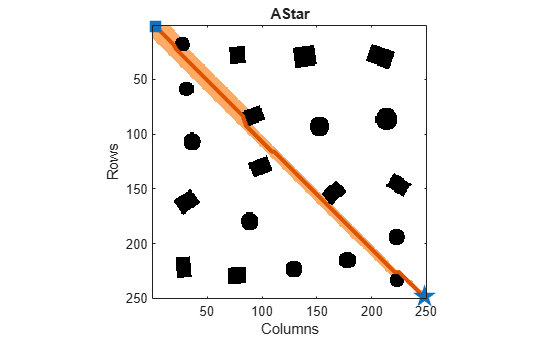# plannerAStarGrid

A* path planner for grid map

## Description

The `plannerAStarGrid` object creates an A* path planner. The planner performs an A* search on an occupancy map and finds shortest obstacle-free path between the specified start and goal grid locations as determined by heuristic cost.

## Creation

### Syntax

``planner = plannerAStarGrid``
``planner = plannerAStarGrid(map)``
``planner = plannerAStarGrid(___,Name,Value)``

### Description

````planner = plannerAStarGrid` creates a `plannerAStarGrid` object with a `binaryOccupancyMap` object using a width and height of 10 meters and grid resolution of 1 cell per meter.```

example

````planner = plannerAStarGrid(map)` creates a `plannerAStarGrid` object using the specified map object `map`. Specify `map` as either a `binaryOccupancyMap` or `occupancyMap` object. The `map` input sets the value of the Map property.```
````planner = plannerAStarGrid(___,Name,Value)` sets Properties using one or more name-value pairs. Unspecified properties have default values. Enclose each property name in quotes.For example, `plannerAStarGrid(map,'GCost','Manhattan')` creates an A* path planner object using the Manhattan cost function.```

## Properties

expand all

Map representation, specified as either a `binaryOccupancyMap` or `occupancyMap` object. This object represents the environment of the robot as an occupancy grid. The value of each grid cell indicates the occupancy of the associated location in the map.

Example: ```planner.Map = binaryOccupancyMap(zeros(50,50));```

The general cost of moving between any two points in a grid, specified as one of the following predefined cost functions `'Chebyshev'`, `'Euclidean'`, `'EulideanSquared'`, or `'Manhattan'`.

Note

You can either use the predefined cost functions or a custom cost function. To use a custom cost function, see GCostFcn property.

Example: `planner = plannerAStarGrid(map,'GCost','Manhattan');`

Example: `planner.GCost = 'Chebyshev';`

Data Types: `string` | `char`

Custom GCost function, specified as a function handle. The function handle must accept two pose inputs as a [row column] vectors and return a scalar of type double.

Note

You can either use the predefined cost functions or a custom cost function. To use the predefined cost functions, see GCost property.

Example: ```planner = plannerAStarGrid(map,'GCostFcn',@(pose1,pose2)sum(abs(pose1-pose2),2));```

Example: ```planner.GCostFcn = @(pose1,pose2)sum(abs(pose1-pose2),2);```

Data Types: `function_handle`

The heuristic cost between a point and the goal in a grid, specified as one of the following predefined cost functions `'Chebyshev'`, `'Euclidean'`, `'EulideanSquared'`, or `'Manhattan'`.

Note

You can either use the predefined cost functions or a custom cost function. To use a custom cost function, see HCostFcn property.

Example: `planner = plannerAStarGrid(map,'HCost','Manhattan');`

Example: `planner.HCost = 'Chebyshev';`

Data Types: `string` | `char`

Custom HCost function, specified as a function handle. The function handle must accept two pose inputs as a [row column] vectors and return a scalar of type double.

Note

You can either use the predefined cost functions or a custom cost function. To use the predefined cost functions, see HCost property.

Example: ```planner = plannerAStarGrid(map,'HCostFcn',@(pose1,pose2)sum(abs(pose1-pose2),2));```

Example: ```planner.HCostFcn = @(pose1,pose2)sum(abs(pose1-pose2),2);```

Data Types: `function_handle`

Toggle tiebreaker mode, specified as either `'on'` or `'off'`.

When you enable the `TieBreaker` property, the A* path planner chooses between multiple paths of the same length by adjusting the heuristic cost value.

Example: `planner = plannerAStarGrid(map,'TieBreaker','on');`

Example: `planner.TieBreaker = 'off';`

Data Types: `string` | `char`

## Object Functions

 `plan` Find shortest obstacle-free path between two points `show` Plot and visualize A* explored nodes and planned path

## Examples

collapse all

Plan the shortest collision-free path through an obstacle grid map using the A* path planning algorithm.

Generate a `binaryOccupancyMap` object with randomly scattered obstacles using the `mapClutter` function.

```rng('default'); map = mapClutter;```

Use the map to create a `plannerAStarGrid` object.

`planner = plannerAStarGrid(map);`

Define the start and goal points.

```start = [2 3]; goal = [248 248];```

Plan a path from the start point to the goal point.

`plan(planner,start,goal);`

Visualize the path and the explored nodes using the `show` object function.

`show(planner)`